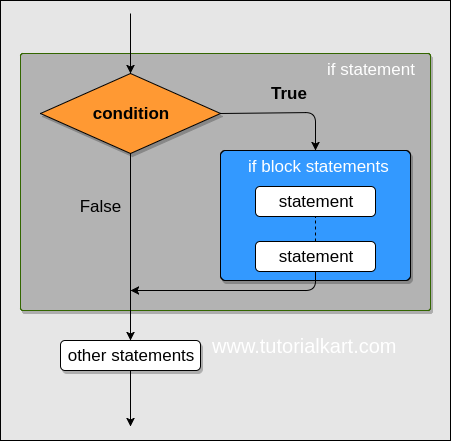## Python If Conditional Statement

Python If Conditional Statement is used to execute a set of statements (present inside if block) when a specific condition is satisfied. If the condition is not satisfied, the statements in the if block are not executed.

In this tutorial, we will learn the syntax of Python If Conditional statement, and how to write if statements in Python program, using some example scenarios.

### Syntax – Python IF

```if <condition> :
statements()```

A pictorial representation of the working is given below :Flowchart of Python If Conditional Statement

### Example Program – Python If

In this example, we will write an if statement with the condition that the product of the two numbers `number_1` and `number_2` is equal to 30.

example.py – Python Program

```number_1 = 5
number_2 = 6

# observe the indentation for the statements in the if block
if number_1 * number_2 == 30:
print('Hello World!')
print('The product of two numbers is 30.')```
Try Online

Output

```Hello World!
The product of two numbers is 30.```

Note : Observe the indentation or alignment of the statements inside the if-block. Unlike programming languages like Java or C where the statements are enclosed in curly braces, python considers the alignment of statements as block representation.

If the alignment is missed out as shown in the below program, interpreter throws IndentationError.

example.py – Python Program

```number_1 = 5
number_2 = 6

# observe the indentation for the statements in the if block
if number_1 * number_2 == 30:
print("The condition is true.")
print("Inside if condition block")
print("statement in if loop")

print("Outside if condition block.")```
Try Online

Output

```File "example.py", line 7
print("Inside if condition block")
^
IndentationError: unexpected indent```

### Python If – Check if Number is Even

In this example, we will take an integer in variable `n`, and check if `n` is even or odd using if statement in Python.

The condition for our if statement would be to check if `n` leaves a reminder of zero when divided by `2`.

example.py – Python Program

```n = 6

if n%2 == 0:
print(f'{n} is even number.')```
Try Online

Output

`6 is even number.`

In the same program, we can check if `n` is odd using another if statement as shown in the following.

example.py – Python Program

```n = 5

if n%2 == 0:
print(f'{n} is even number.')

if n%2 != 0:
print(f'{n} is odd number.')```
Try Online

Output

`5 is odd number.`

Since n is 5, the condition `n%2 == 0` evaluates to false and the statement inside first if statement is not executed. Whereas in the second if statement, the `n%2 != 0` evaluates to true for `n=5`. Therefore, the statement(s) inside second if block were executed.

### Conclusion

In this Python Tutorial, we have seen the syntax of if statement in python, a pictorial representation of working of if statement, an example and how the indentation of the statements in an if block should be, and IndentationError if we miss out indentation.